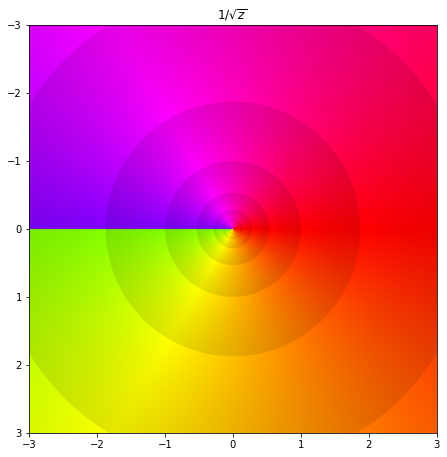# Holomorphic functions#

## Complex differentiation#

Holomorphic functions (often called complex analytic functions)

A function $$f : D \to \mathbb{C}$$, where $$D \subseteq \mathbb{C}$$ is an open set, is complex-differentiable at a point $$z_0 \in D$$ if there exists

$f'(z_0) = \lim_{z \to z_0} \frac{f(z) - f(z_0)}{z - z_0} \in \mathbb{C}.$

If $$f : D \to \mathbb{C}$$ is differentiable at all points of $$D$$, we say it is holomorphic (often analytic) in $$D$$. If a function is holomorphic in $$\mathbb{C}$$ we say that it is entire.

Examples:

• $$z$$ is entire

• all polynomials are entire

• $$1/z$$ is holomorphic in $$\mathbb{C} \backslash { 0 }$$

• all rational functions are holomorphic in $$\mathbb{C}$$ except in the zeros of their denominators

• $$\sin z, \cos z$$ are entire

• $$\sqrt{z}$$ is holomorphic in $$\mathbb{C} \backslash (- \infty, 0]$$

import numpy as np
import matplotlib.pyplot as plt
from matplotlib.colors import hsv_to_rgb

def phaseplot(ax, f, x=None, y=None):

a = np.log(np.abs(f)) * 20 / (2*np.pi)
a = a - a//1
_amin = np.min(a)
_amax = np.max(a)

h = (np.angle(-f) + np.pi) / (2*np.pi)
v = (a - _amin) / (_amax - _amin) * (1 - 0.9) + 0.9

if x is None:
extent = None
else:
extent = [min(x), max(x), min(y), max(y)]

img = hsv_to_rgb(np.dstack((h, np.ones(f.shape), v)))

ax.imshow(img, extent=extent)
ax.invert_yaxis()

x = np.linspace(-3, 3, 1000)
X, Y = np.meshgrid(x, x)
Z = X + 1j*Y

fig = plt.figure(figsize=(7.5, 7.5))
ax.set_title('$1/ \sqrt{z}$')

phaseplot(ax, 1/np.sqrt(Z), x, x)
plt.show()If we go back to thinking about a derivative of a real function of one variable, recall that for the derivative to exist at $$x_0$$ the limit when approaching $$x_0$$ from the left and from the right must be equal to each other. That is because $$x_0$$ is just a number on the number line. Complex numbers, however, lie on the plane, so we can approach some complex number $$z_0$$ from any direction. Therefore, the limit above must exist for any angle of approach to $$z_0$$ and it must always be the same. This is a very strict condition!

For $$f$$ to be differentiable at $$z_0$$ it must be continuous at $$z_0$$. That means that

$\lim_{z \to z_0} f(z) = f(z_0)$

for finite $$z_0, f(z_0)$$. Recall that we can separate the real and imaginary parts of a complex function: $$f(z) = u(x, y) + iv(x, y)$$. For $$f$$ to be continuous, it is enough to show that $$u$$ and $$v$$ are continuous. If $$f$$ is holomorphic on $$D$$ then it is continuous on $$D$$.

Differentiation rules

The addition, product, quotient and chain rules apply in complex differentiation similarly to real calculus. Let $$f, g : D \to \mathbb{C}$$ be differentiable at $$z_0 \in D$$, then:

• $$\alpha f + \beta g$$ is differentiable for all $$\alpha, \beta \in \mathbb{C}$$ at $$z_0$$: $$(\alpha f + \beta g)’(z_0) = \alpha f’ (z_0) + \beta g’(z_0)$$

• $$fg$$ is differentiable at $$z_0$$: $$(fg)'(z_0) = f'(z_0)g(z_0) + f(z_0)g'(z_o)$$

• $$\frac{f}{g}$$ is differentiable at $$z_0$$ for $$g(z_0) \neq 0$$: $$\left( \frac{f}{g} \right)’ (z_0) = \frac{f’(z_0)g(z_0) - f(z_0)g’(z_0)}{(g(z_0))^2}$$

• Let $$h: D_1 \to \mathbb{C}$$, where $$D_1$$ is an open set containing $$f(D)$$. If $$h$$ is differentiable at $$f(z_0)$$, then $$h \circ f$$ is differentiable at $$z_0$$: $$(h \circ f)’(z_0) = h’(f(z_0))f’(z_0).$$

## Cauchy-Riemann conditions#

Cauchy-Riemann theorem. A complex function $$f = u + iv : D \to \mathbb{C}$$ is differentiable at a point $$z_0 = (x_0, y_0)$$ iff the real-valued functions of two real variables $$u$$ and $$v$$ are differentiable at a point $$(x_0, y_0)$$ and they satisfy the Cauchy-Riemann conditions:

$\partial_x u = \partial_y v, \qquad \partial_y u = - \partial_x v.$

This is a fundamental result in complex analysis - a necessary and sufficient condition for any complex function to be differentiable. Furthermore,

\begin{split} \begin{aligned} f'(z_0) & = \partial_x u + i \partial_x v =: \partial_x f(z_0) \\ & = \partial_x u - i \partial_y u \\ & = \partial_y v - i \partial_y u =: -i \partial_y f(z_0) = \frac{1}{i} \partial_y f(z_0) = \partial_{iy} f(z_0) \\ & = \partial_y v + i \partial_x v. \end{aligned} \end{split}

If Cauchy-Riemann conditions are satisfied, then $$u$$ and $$v$$ are harmonic functions, i..e they are a solution to the Laplace equation:

$u_{xx} + u_{yy} = 0.$

Examples: basic derivatives

$(z^n)' = nz^{n-1}$
\begin{split} \begin{aligned} \exp' z & = (e^x(\cos y + i \sin y))' \\ & = \partial_x u(z) + i \partial_x v(z) = \partial_x (e^x \cos y) + i \partial_x (e^x \sin y) = e^x \cos y + i e^x \sin y \\ & = \exp z \end{aligned}\end{split}